# AP Chemistry : Elemental Composition of Pure Substances

## Example Questions

### Example Question #1 : Help With Lewis Diagrams

Which of the following compounds breaks the octet rule?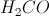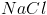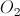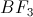Explanation:

The octet rule requires that each atom in the molecule has eight valence electrons, fully filling the s and p subshells. Atoms that have a full octet tend to be more stable and lower in energy.

In the compound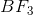, each fluorine has seven initial valence electrons and boron has three initial valence electrons. Upon forming the compound bonds, boron shares its three electrons with each fluorine through covalent bonds. This givens each fluorine a full octet, but leave boron with only three covalent bonds, resulting in only six valence electrons. Boron does not satisfy the octet rule in this compound.

Each atom in the other listed compounds forms bonds to generate a full octet. Sodium chloride achieves this through an ionic bond. Methanal and diatomic oxygen both use double bonds to help achieve octets.

### Example Question #21 : Elemental Composition Of Pure Substances

The molecular formula of a compound __________.

is usually a multiple of the empirical formula

is impossible to determine

is constant regardless of the compound

is not related to the empirical formula

is usually a multiple of the empirical formula

Explanation:

The empirical formula of a compound is the simplest whole number ratio of the elements in the compound, whereas the molecular formula gives the exact number of atoms of each element in the compound—this is usually a multiple of the empirical formula. If the empirical formula of a compound is CH, a common molecular formula is C6H6 (benzene).

### Example Question #22 : Elemental Composition Of Pure Substances

What is the molecular formula for Cobalt (II) Nitrate?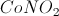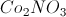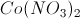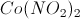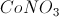Explanation:

Cobalt (II) is Cobalt in its second oxidation state,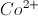, and the chemical formula for Nitrate is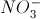. The neutral salt is.

### Example Question #671 : Ap Chemistry

What is the molecular formula for ferrous sulfide?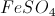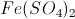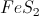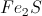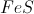Explanation:

Ferrous is the name for Iron (II), or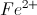. Sulfide is the anion of sulfur,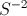. The chemical formula for ferrous sulfide is.

### Example Question #21 : Elemental Composition Of Pure Substances

The periodic table of elements is organized in order of atomic number, whic describes how many protons are found in the atom's nucleus. The position of the element in the periodic table can also yield insight into how that atom will respond to other atoms and how it will participate in a chemical reaction.

Which of the following chemical compounds shows the correct composition of sodium and oxygen?

Na3O

NaO

Na2O3

NaO2

Na2O

Na2O

Explanation:

Remember that sodium wants to lose only one electron in order to achieve a stable octet. Meanwhile, oxygen needs to gain 2 electrons in order to achieve a stable octet. As a result, the expected compound involves two sodium atoms, each giving up one electron in order to fill oxygen's octet.

### Example Question #1 : Naming Compounds

Which answer choice shows the correct chemical formula for barium sulfate?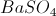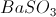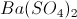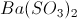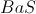Explanation:

We should first remember the difference between sulfate, sulfite, and sulfide. Sulfate is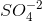, sulfite is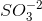` and sulfide is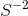.

The only answer choices that could be right must havein them. We then need to see that barium usually has a charge of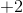, as the periodic table shows us, and so we need a charge ofto cancel that out. The answer is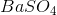.

### Example Question #673 : Ap Chemistry

What is the IUPAC name for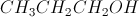?

Propanol

Ethanol

Propenol

Propane

Propene

Propanol

Explanation:is a saturated hydrocarbon with an organic functional group,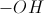, or alcohol.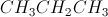is propane, so we will remove the "e" from the end of that name and add the suffix "ol" to denotate an alcohol, so the correct answer is propanol.

Propenol is propanol with a double bond in the hydrocarbon chain. Ethanol is a saturated two-carbon chain with an alcohol functional group.

### Example Question #22 : Elemental Composition Of Pure Substances

Name the following compound: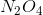.

Nitrogen(IV) tetraoxide

Dinitrogen tetraoxide

Nitride oxide

Dinitrogen tetraoxide

Explanation:

Covalent non-metal/non-metal compounds are named by taking the name of each element and appending the apropriate greek prefix to indicate the number of each atom present—in this case, di- for two and tetra- for four. The end of the more electronegative element is substituted with "ide". This gives the name of dinitrogen tetraoxide.

### Example Question #21 : Elemental Composition Of Pure Substances

What is the systematic name for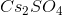?

Cesium (I) sulfate

Cesium sulfite

Cesium sulfate

Cesium sulfur tetraoxide

Cesium sulfate

Explanation:

Ionic compounds are named by naming the cation followed by the anion. Since the cation, cesium, is an alkali metal the oxidation state, should not be specified. Alkali metals will always have an oxidation state of 1. The anion,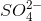, is sulfate. Together, the full name of the compound is cesium sulfate.

### Example Question #25 : Elemental Composition Of Pure Substances

Name the following compound: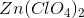Zinc chloride

Zinc chlorate

Zinc dichlorate

Zinc chloride tetroxide

Zinc perchlorate

The compound's name is zinc perchlorate. With the addition of a fourth oxygen atom to the molecule, chlorate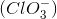, we follow the polyatomic ion naming rules and add a per- to the molecule's name, giving us perchlorate. When bonded ionically to a zinc cation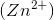, the resulting ionic compound is called zinc perchlorate.